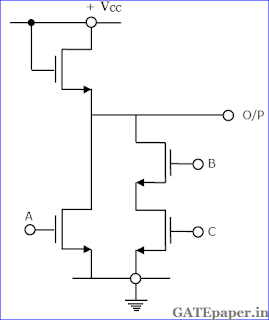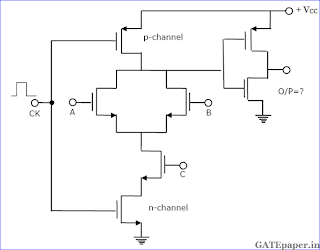### GATE 1991 ECE Video Solutions on Digital Circuits (Digital Electronics)

1. Two dimensional addressing of 256 X 8 bit ROM using 8 to 1 selectors requires _______ (how many?) NAND gates.

2. The CMOS equivalent of the following nMOS gate (shown in figure) is ________ (Draw the circuit).3. A bit stored in a FAMOS device can be erased by ___________.

4. In the figure, the Boolean expression for the output in terms of inputs A, B and C when the clock CK is high, is given by ____________5. The program given below is run on an 8085 based microcomputer system. Determine the contents of the registers: PC, SP, B, C, H, L after a halt instruction is executed.Solution:

6. The four variable function f is given in terms of min-terms as f(A, B, C, D) = ∑m(2,3,8,10,11,12,14,15). Using the K-map minimize the function in the sum of products form. Also, give the realization using only two input NAND gates.

7. An S-R flip flop can be converted into a T flip flop by connecting ____ to Qbar and _____ to Q.

#### 1 comment:

1.link of solution of 5th question

Name

Email *

Message *